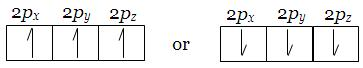1800-1023-196

+91-120-4616500

# Important Rules for Filling Orbital

## Important Rules for Filling Orbital

### (1) Aufbau’s principle

This principle states that the electrons are added one by one to the various orbitals in order of their increasing energy starting with the orbital of lowest energy. The increasing order of energy of various orbitals is

1s<2s<2p<3s<3p<4s<3d<3d<5s<5p<6s<4f<5d<6p<7s<5f<6d<7p……

### (2) (n+l) Rule

In neutral isolated atom, the lower the value of (n + l) for an orbital, lower is its energy. However, if the two different types of orbitals have the same value of (n + l), the orbitals with lower value of n has lower energy.

### (3) Pauli’s exclusion principle

According to this principle “no two electrons in an atom will have same value of all the four quantum numbers”.

If one electron in an atom has the quantum numbers n = 1, l=0, m = 0, and s = +1/2, no other electron can have the same four quantum numbers. In other words, we cannot place two electrons with the same value of s in a 1s orbital.

The orbital diagram does not represent a possible arrangement of electrons

Because there are only two possible values of s, an orbital can hold not more than two electrons.

### (4) Hund’s Rule of maximum multiplicity

This rule deals with the filling of electrons in the orbitals having equal energy (degenerate orbitals). According to this rule, “Electron pairing in p, d and f orbitals cannot occur until each orbitals of a given subshell contains one electron each or is singly occupied”.

This is due to the fact that electrons being identical in charge, repel each other when present in the same orbital. This repulsion can however be minimized if two electrons move as far apart as possible by occupying different degenerate orbitals. All the unpaired electrons in a degenerate set of orbitals will have same spin.

As we now know the Hund’s rule, let us see how the three electrons are arranged in p orbitals.

The important point to be remembered is that all the singly occupied orbitals should have electrons with parallel spins i.e in the same direction either-clockwise or anticlockwise.## NEET & AIIMS Exam Sample Papers

 AIIMS SAMPLE PAPERS View More NEET SAMPLE PAPERS View More